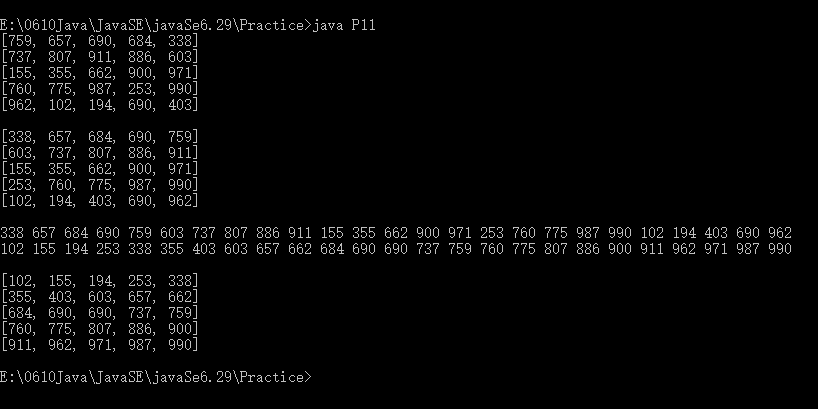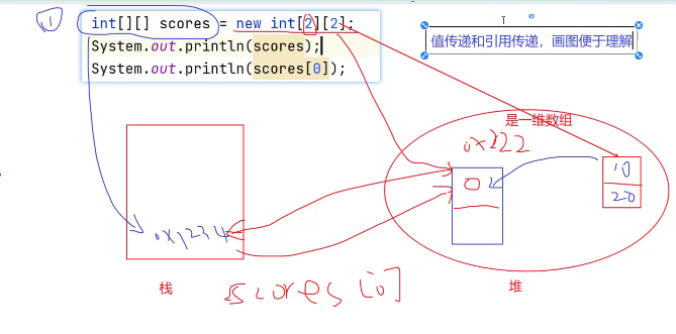• ## Java二维数组排序

万次阅读 2019-06-29 15:46:12
Java二维数组排序


Java二维数组排序
Java二维数组排序

Java二维数组排序
关于Java二维数组的排序方法之一是把二维数组放进一维数组然后试用版Arrays.sort();进行排序，排序结束后再把一维数组内容重新写入二维数组内，代码实现如下：
为了方便，我在这里使用了随机数生成方法Math.random()进行随机数生成，依次写入二维数组内：
import java.util.*;
public class P11{
public static void main(String[] args){
int[][] arr=new int;
for(int i=0;i<arr.length;i++){
for (int j=0;j<arr[i].length;j++){//生成随机数【100-1000】
arr[i][j]=(int)(Math.random()*(1000-100+1)+100);
}
}
for(int i=0;i<arr.length;i++){
System.out.println(Arrays.toString(arr[i]));
}
System.out.println();
for(int i=0;i<arr.length;i++){
Arrays.sort(arr[i]);
}
for(int i=0;i<arr.length;i++){
System.out.println(Arrays.toString(arr[i]));
}
int[] temp=new int;
int k=0;
for(int i=0;i<arr.length;i++){
for(int j=0;j<arr[i].length;j++){
temp[k]=arr[i][j];
k++;
}
}
System.out.println();
for(int x:temp){
System.out.print(x+" ");
}
Arrays.sort(temp);
System.out.println();
for(int x:temp){
System.out.print(x+" ");
}
k=-1;
for(int i=0;i<arr.length;i++){
for(int j=0;j<arr[i].length;j++){
k++;
arr[i][j]=temp[k];
}
}
System.out.println();
System.out.println();
for(int i=0;i<arr.length;i++){
System.out.println(Arrays.toString(arr[i]));
}
}
}

因为使用了随机数生成的数组内容，所以每次运行数组内容都不尽相同，本次运行结果如下：展开全文二维数组 排序
• 维数组排序很简单，调用Arrays.sort(array)就可以直接进行排序。二维数组稍微复杂一些。 二维数组根据第一列排序： int[][] ts_id = new int[][] {{1,2},{99,0},{876,9},{3,100}}; Arrays.sort(ts_.
原文链接：https://blog.csdn.net/qq_43220949/article/details/109037667
写Leetcode第435题需要用到对二维数组的按列排序（对第二列），特此记录。
一维数组排序很简单，调用Arrays.sort(array)就可以直接进行排序。二维数组稍微复杂一些。

二维数组根据第一列排序：
int[][] ts_id = new int[][] {{1,2},{99,0},{876,9},{3,100}};

Arrays.sort(ts_id,new Comparator<int[]>() {
public int compare(int[] o1, int[] o2) {
return o1-o2;
}
});
其实也是用的api，使用Arrays.sort（array,new Comparator(){}）
传入比较器Comparator，比较器内部需要实现compare(o1,o2)方法，方法内部就是具体的排序细节。
比如：二维数组根据第一列排序，就让o1 -o2,如果用 o1-o2 ,即升序；反之 o2-o1 就降序。【o1就代表取第一列。】
还可以有很多拓展，比如如果第一列相同，就根据第二列排序：
Arrays.sort(ts_id,new Comparator<int[]>() {
@Override
public int compare(int[] o1, int[] o2) {
if (o1 == o2) {
return o1 - o2;
}
return o1-o2;
}
});

展开全文• 二维数组 int[][] 数组名=new int; import java.util.Scanner; public class Day6T1 { public static void main(String[] args) { int[][] scores = new int[]; Scanner input = new Scanner(System...
二维数组
int[][] 数组名=new int;
import java.util.Scanner;
public class Day6T1 {
public static void main(String[] args) {
int[][] scores = new int[];
Scanner input = new Scanner(System.in);
for (int i = 0; i < scores.length; i++) {
int sum = 0;    //计算每个班级的总分
System.out.println("输入第"+(i+1)+"个班级的人数：");
int num=input.nextInt();
System.out.println("输入第"+(i+1)+"个班级");
scores[i]=new int[num];
//在每行，对每个单元格填充数值
for (int j = 0; j < num; j++) {
System.out.println("输入第"+(j+1)+"个学生的成绩:");
scores[i][j] = input.nextInt();
sum += scores[i][j];
}
System.out.println("第"+(i+1)+"个班级的总分为:" + sum);
System.out.println("第"+(i+1)+"个班级的平均分为:" + sum/num);
}
}
}冒泡排序
public class BubbleSort {

public static void bubbleSort(int[] data) {
System.out.println("开始排序");
int arrayLength = data.length;
//外层循环控制轮数
for (int i = 0; i < arrayLength - 1; i++) {
for (int j = 0; j < arrayLength - 1 - i; j++) {
//涉及到交换位置
if (data[j] > data[j + 1]) {
int temp = data[j + 1];
data[j + 1] = data[j];
data[j] = temp;
}
}
//每一轮比较完之后，输出结果
//System.out.println(Arrays.toString(data));
}
}

插入排序

## 快速排序
## 选择排序
## 堆排序


展开全文• //定义一个二维数组，其中所包含的一维数组具有两个元素 对于一个已定义的二位数组a进行如下规则排序,首先按照每一个对应的一维数组第一个元素进行升序排序（即a[]）,若第一个元素相等,则按照第二个元素进行升序...
int [][]a = new int ;
//定义一个二维数组，其中所包含的一维数组具有两个元素
对于一个已定义的二位数组a进行如下规则排序,首先按照每一个对应的一维数组第一个元素进行升序排序（即a[]）,若第一个元素相等,则按照第二个元素进行升序排序（a[]）。(特别注意,这里的a[]或者a[]在java中是不能这么定义的,这里只是想说明是对于某一个一维数组的第0或1个元素进行排序)
Arrays.sort(a, new Comparator<int[]>() { @Override public int compare(int[] o1, int[] o2) { if (o1==o2) return o1-o2; return o1-o2; } }); 其中o1-o2表示对于第二个元素进行升序排序如果为o2-o1则表示为降序。
展开全文• 排序规则: 先按数组的第一个元素进行升序排序，若第一个元素相等,则按照第个元素进行升序排序。 使用API：Arrays.sort()(T[] a, Comparator<? super T> c)，该API根据给定的比较器(设定排序方式)对指定的...
• ◆◆◆一、理解一维数组的定义和应用，了解二维数组和控件数组；1、数组的概念：数组并不是一种数据类型，而是一组相同类型数据的集合。用一个统一的名字(数组名)代表逻辑上相关的一批数据，每个元素用下标变量来...
• ***解题思路***将二维数组抽调成一维数组，再又一维数组还原成二维数组 import java.util.Arrays;//引入Arrays类 public class test {public static void main(String[] args) { int array[][]={{11,4,2},{19,21,1}...
• 二维数组排序，按照某一列列大小对数组进行排序。二维数组的排序直接调用Arrays.sort(); 虽然不会出现编译错误，但运行时会出现以下错误提示： 说明不可以这样用，而我们又想对它排序，就可以使用Comparator来外部...
• 文章目录方法一：重写Arrays.sort方法方法：...待排序数组形式int[][] array = new int[n]，其中n为任意整数。 import java.util.Arrays; public class Sort { public static int[][] mySort(int[][]...sort 排序算法
• //借助Arrays的方法对二维数组进行排序。 //参考思路： //先把二维数组使用System.arraycopy进行数组复制到一个一维数组 //然后使用sort进行排序 //最后再复制回到二维数组。 import java.util.Arrays; public ...
• java动态创建二维数组,从零学java笔录-第31篇 图解二位数组在内存中存储,java二维数组动态赋值,java二维数组创建方法二维数组的定义 type arrayName[ ][ ]; type [ ][ ]arrayName Java 二维数组的声明、初始化和引用...
• 借助Arrays的方法对二维数组进行排序。 参考思路： 先把二维数组使用System.arraycopy进行数组复制到一个一维数组 然后使用sort进行排序 最后再复制回到二维数组。 代码示例 package Test; import java.lang....算法
• 二维数组中（每个一维数组的长度相同），按照如下规则排序：每一行都按照从左到右递增的顺序排序，每一列都按照从上到下递增的顺序排序 public class HashMapTest { public static void main(String[] args) { ...数组排序
• 运用java 实现二维数组排序 （排序要求为‘基于第一个元素排序’组合不变） 三种排序方法分别为： 选择排序，插入排序和排列排序； //选择排序 public class Sort { public static void main(String[] args) { int...算法
• 假设有个二维数组： int[][] arr = {{1,2},{3,4},{5,6}}; 需要给按一定规则调整，其中一维数组的顺序，比如规则为按一维数组的首元素从小到大排。 数组排序可以使用Arrays.sort()，自定义规则的话需要自己定义...
• 排序规则：首先按照每个一维数组第一个元素进行升序排序,若第一个元素相等,则按照第个元素进行升序排序。 原代码 Arrays.sort(a, new Comparator<int[]>() { @Override public int compare(int[] o1, int...数据结构 算法 c++
• 以前都是将二维转换成一维数组排序，今天老师要求不转换试了一下，仔细分析了一下冒泡，写了这个大家可以试试其他的排序方法，应该都可以用 import java.util.Arrays; class Test { public static void main...
• Java 二维数组按指定列排序 简介： 在做项目时，需要对一个二维数组，按照指定的列进行排序。 效果图： 代码实现： public static void main(String[] args) { int[][] nums = initialArray(5, 3); System.out....
• 利用冒泡排序对字符串二维数组进行排序 package lan; public class array { public static void main(String[] args) { String arr[][]= {{"青年节","5.4"}, {"教师节","9.10"}, {"女生节","3.9"}, {"妇女...字符串
• Java 数组排序，数组内存储整形数，前一列先排，排完再在前列的基础上再排，同一行的数不会再排序时变位置，跟excel表格排序一样，使用冒泡排序。
• Java 二维数组按指定列排序（二） 上一篇文章【Java 二维数组按指定列排序（一）】，我们介绍了二维数组按列排序的方法。本章我们对上次的方法进行修改，使其能够支持不同的排序方式（升序 or 降序）。 效果图： ...
• /** *author：Alex.wen *2017年8月18日下午8:27:56 */ public class shuzumaopaopaixufa { public static void maopao(int[][]a) { int sum=0; for(int i=0;...//求出二维数组元素个数； } int[]a1=new...

# java二维数组排序java 订阅